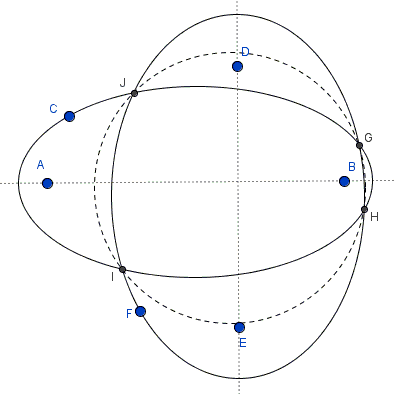# Concyclic Points of Two Ellipses with Orthogonal Axes

In the applet there are two ellipses each defined by its two foci and a point on its boundary. All six are draggable.

### Problem

Given two ellipses with orthogonal axes.If there are four points of intersection, all four are concyclic.

### Hint

Analytic geometry makes a simple but powerful application for this problem. Just choose a suitable system of coordinates. In addition, it makes sense to check various equations that an ellipse can be defined with.

### Solution 1

Ellipse can be defined ad the locus of points whose distance to a given line (called directrix) times a given number from $(0,1)$ equals the distance of the point to a given one (called focus.) Ellipse also has a long axis and a short axis, and the directrix is perpendicular to the long which also house its foci.

So let's choose a Cartesian coordinate system with the axes along the long axes of the two ellipses. Let their foci be respectively at $(\bar{a},0)$ and $(0,\tilde{a}),$ directrices at $y=-\bar{b}$ and $x=-\tilde{b}$ such that one is given by the equation $|(y+\bar{b})\bar{e}|=\sqrt{x^{2}+(y-\bar{a})^{2}}$ while the other is defined by $|(x+\tilde{b})\tilde{e}|=\sqrt{(x-\tilde{a})^{2}+y^{2}},$ where $\bar{e}$ and $\tilde{e}$ are their eccentricities. The four points of intersetion $(x_{i},y_{i}),$ $i=1,2,3,4,$ satisfy both equations. The reason I chose these equations is that a single squaring eliminates all radicals. We thus have

$(y_{i}+\bar{b})^{2}=[x_{i}^{2}+(y_{i}-\bar{a})^{2}]/\bar{e}^{2}$ and $(x_{i}+\tilde{b})^{2}=[(x_{i}-\tilde{a})^{2}+y_{i}^{2}]/\tilde{e}^{2},$

$i=1,2,3,4.$ Each of the four points satisfies both equations and, therefore, the sum of the two. The sum of the two equations is of the second order. I am mostly concerned with the coefficients by $x^2$ and $y^2.$ Taking all the terms to the right, the sum of the two equations is

$x^{2}[(1/\bar{e})^{2} + (1/\tilde{e})^{2} - 1]+ y^{2}[(1/\bar{e})^{2} + (1/\tilde{e})^{2} - 1] +\ldots = 0.$

The coefficients by $x^2$ and $y^2$ are equal and differ from 0, implying that the equation is that of a circle.

### Solution 2

In the first solution I assumed that the directrices of the two ellipses are perpendicular. On closer inspection, this assumption is not necessary. Let there be two ellipses:

$\displaystyle\bar{f}(x,y)=\frac{(x-\bar{u})^2}{\bar{a}^2}+\frac{(y-\bar{v})^2}{\bar{b}^2}-1=0,\space$ and
$\displaystyle\tilde{f}(x,y)=\frac{(x-\tilde{u})^2}{\tilde{a}^2}+\frac{(y-\tilde{v})^2}{\tilde{b}^2}-1=0.$

The plan is to show that there is a linear combination $\alpha\bar{f}+\beta\tilde{f}$ of the two equations that describes a circle. Naturally, our only concern is the equality of the coefficients by $x^2$ and $y^2$. Thus, we seek $\alpha$ and $\beta$ such that

$\displaystyle\frac{\alpha}{\bar{a}^2}+\frac{\beta}{\tilde{a}^2}=\frac{\alpha}{\bar{b}^2}+\frac{\beta}{\tilde{b}^2},$

or,

$\displaystyle\alpha\big(\frac{1}{\bar{a}^2}-\frac{1}{\bar{b}^2}\big)=\beta\big(\frac{1}{\tilde{b}^2}-\frac{1}{\tilde{a}^2}\big).$

Now, if either $\bar{a}^2=\bar{b}^2$ or $\tilde{a}^2=\tilde{b}^2,$ we already have a circle and there is nothing else to prove. Otherwise, we can certainly choose $\alpha$ and $\beta$ to make the equality hold.

### Acknowledgment

This problem was posted by a post by Emmanuel Antonio José García at the CutTheKnotMath facebook page.### Conic Sections > Ellipse